# Zipf’s Law and Subsets of Lexis

Maciej Eder, Rafał L. Górski, Joanna Byszuk

Zipf’s Law and Subsets of Lexis

Maciej Eder, Rafał L. Górski, Joanna Byszuk

Institute of Polish Language (Polish Academy of Sciences)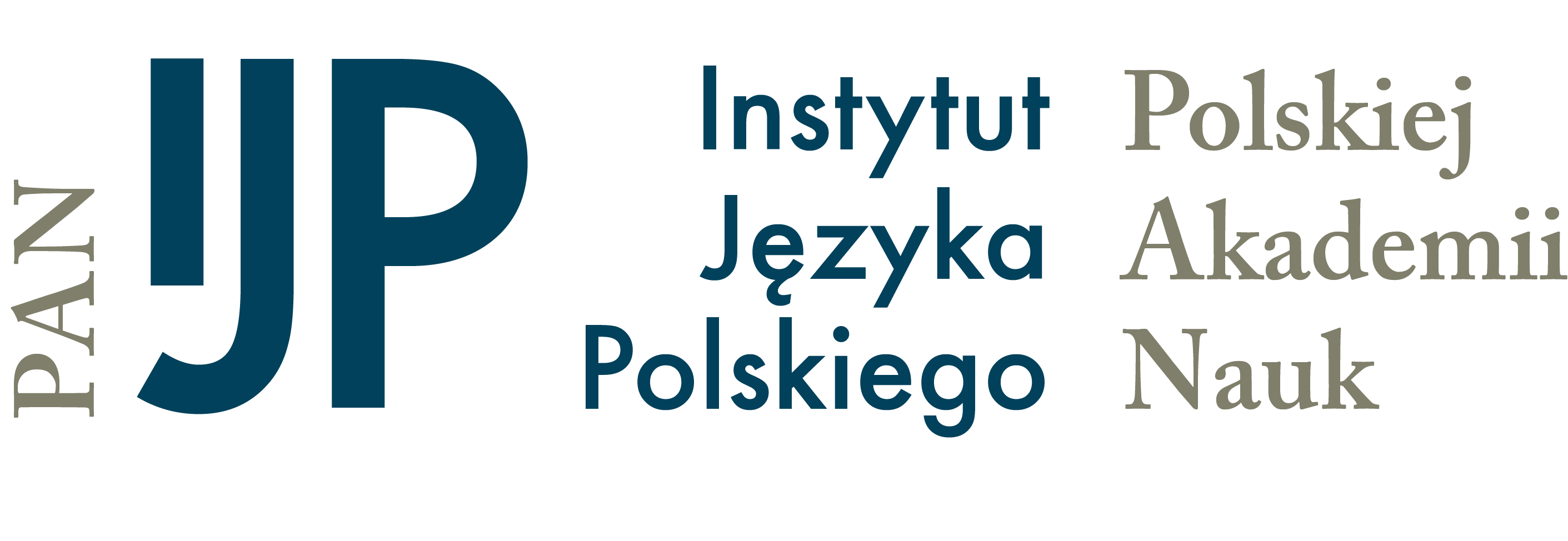Qualico 2018, Wrocław, 5th July 2018

## Zipf’s law and language

• It has been observed that the language is Zipfian.
• However, what is “the language”?
• orthographic forms or lemmata?
• only words or grammatical categories as well?
• unigrams or also n-grams?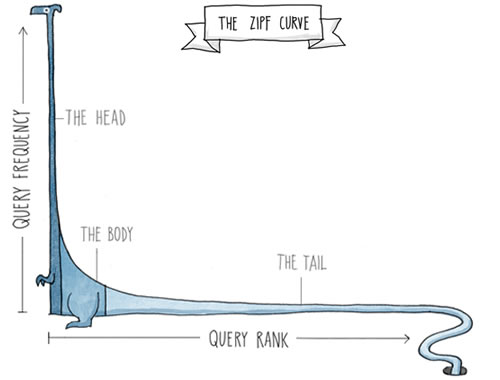## Zipf’s Law on a log-log scale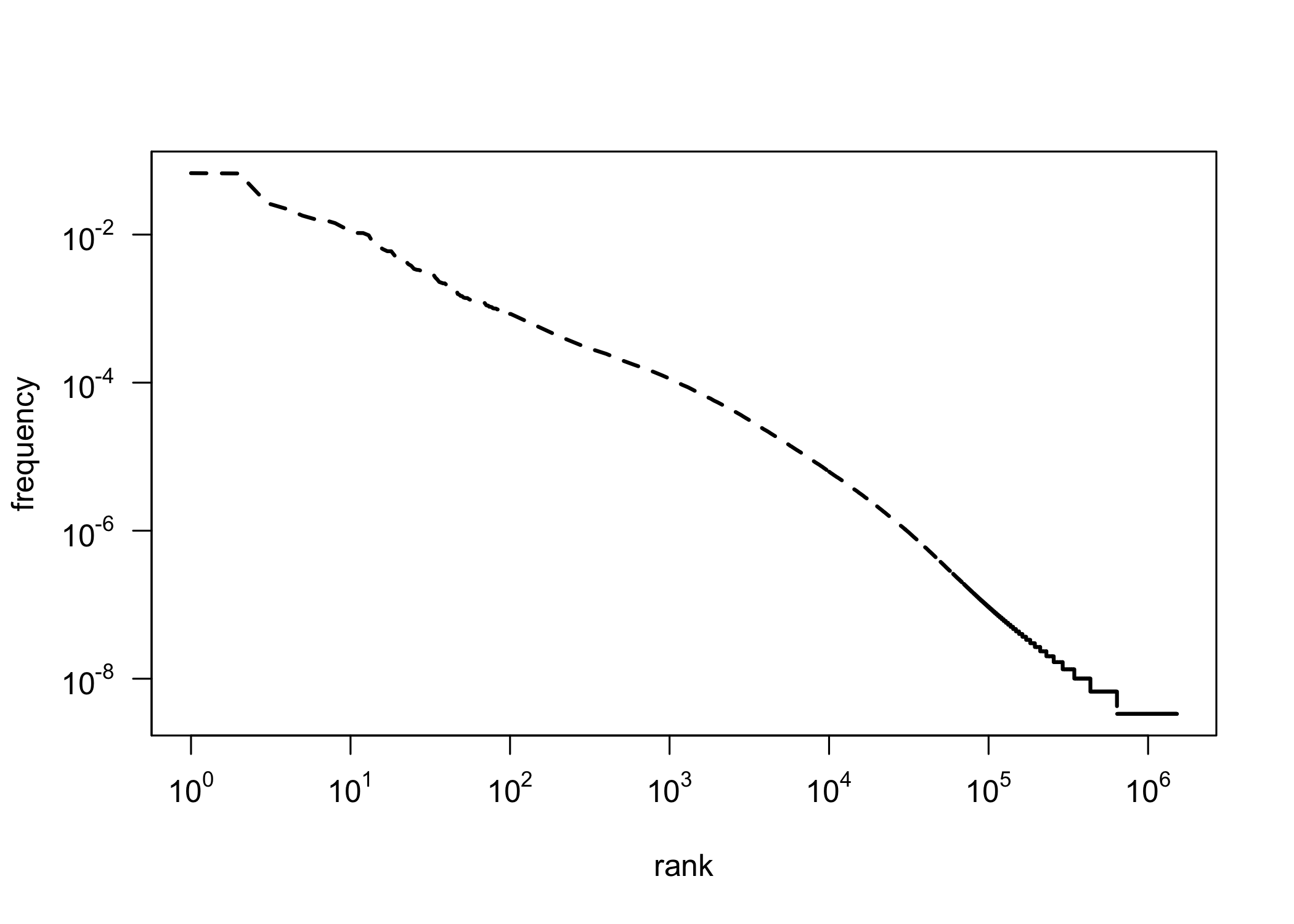## Research question

• If the distribution of all the words in the corpus is Zipfian, is the distribution of a subset of these words also Zipfian?
• Since a text is a sum of nouns, verbs, adjectives, prepositions etc., is the distribution of particular classes (nouns, verbs etc.) also Zipfian?

## Observations on Brown Corpus

[W]ord categories are also fit nicely […] perhaps even more closely than words—but the shape of the fit […] differs.

The general pattern suggests that a full explanation of the word frequency distribution would ideally call on mechanisms general enough to apply to syntactic categories and possibly even other levels of analysis.

## Dataset

• The balanced version of the National Corpus of Polish
• 300 mln segments, i.e. roughly 250 mln words.
• The POS tags and lemmata taken as they are.
• The corpus tagged automatically, using a 1 mln manually tagged subset.
• Consequently, some wrongly assigned tags should be expected!

## Categories

• Very granular parts of speech in the National Corpus of Polish.
• For that reason, we split the tags:
• the proper POS: subst, adj, fin, prep, …
• the number: sg, pl
• the case: nom, gen, dat, …
• the person: 1st, 2nd, 3rd (not included in a tag)
• Independently, we tested POS-tag n-grams

## First observations: singular vs. plural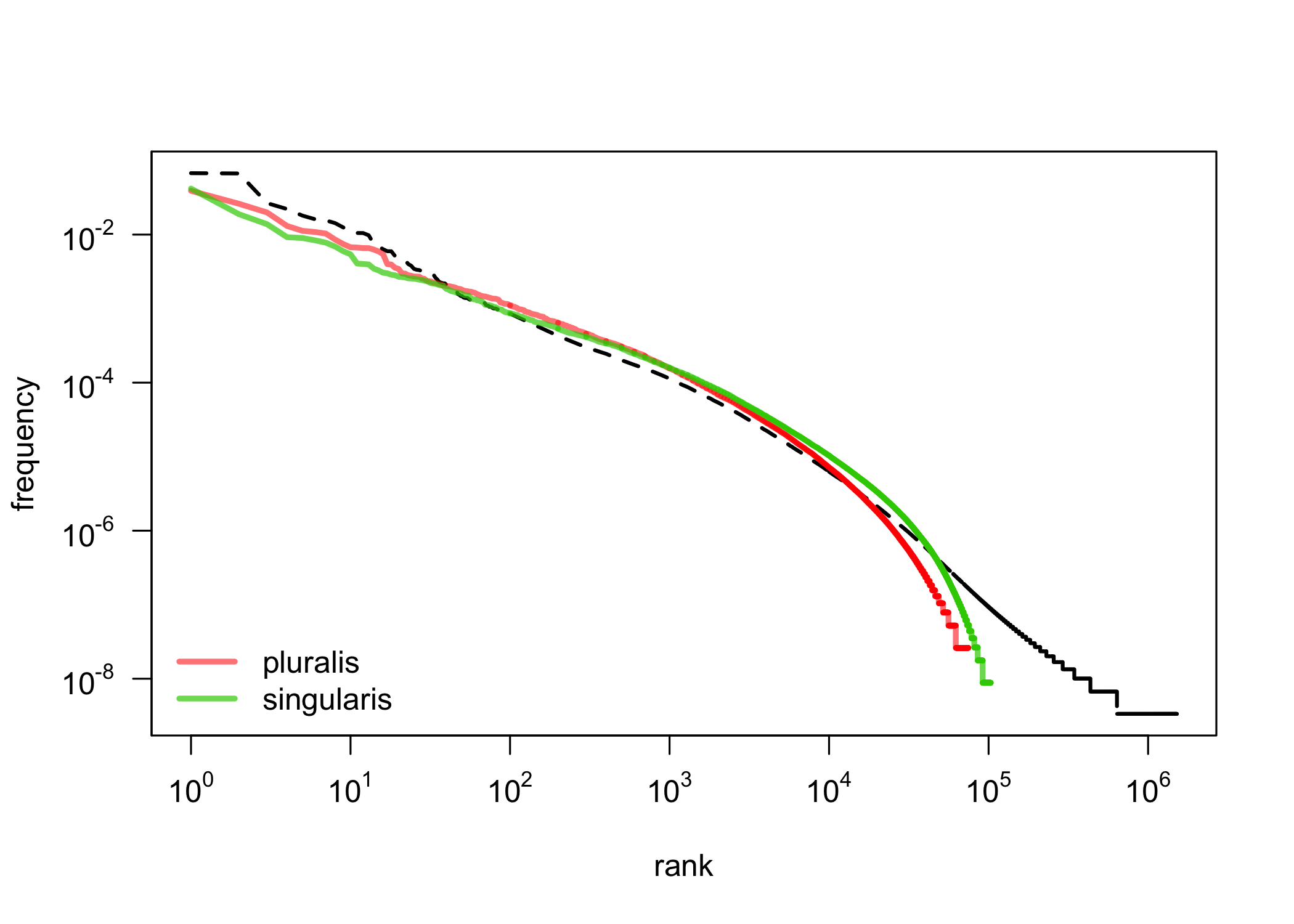## First observations: 1st, 2nd, 3rd person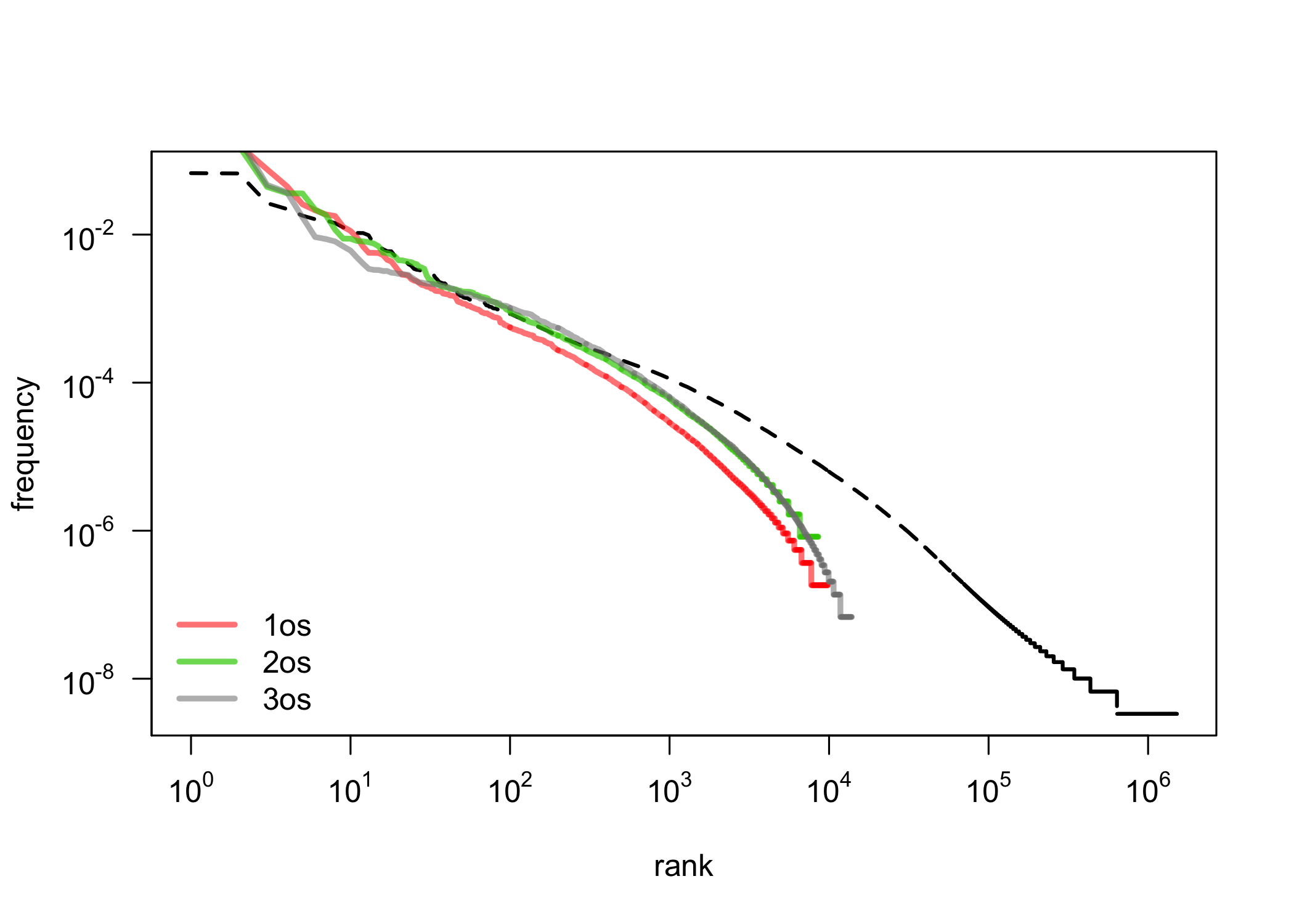## First observations: cases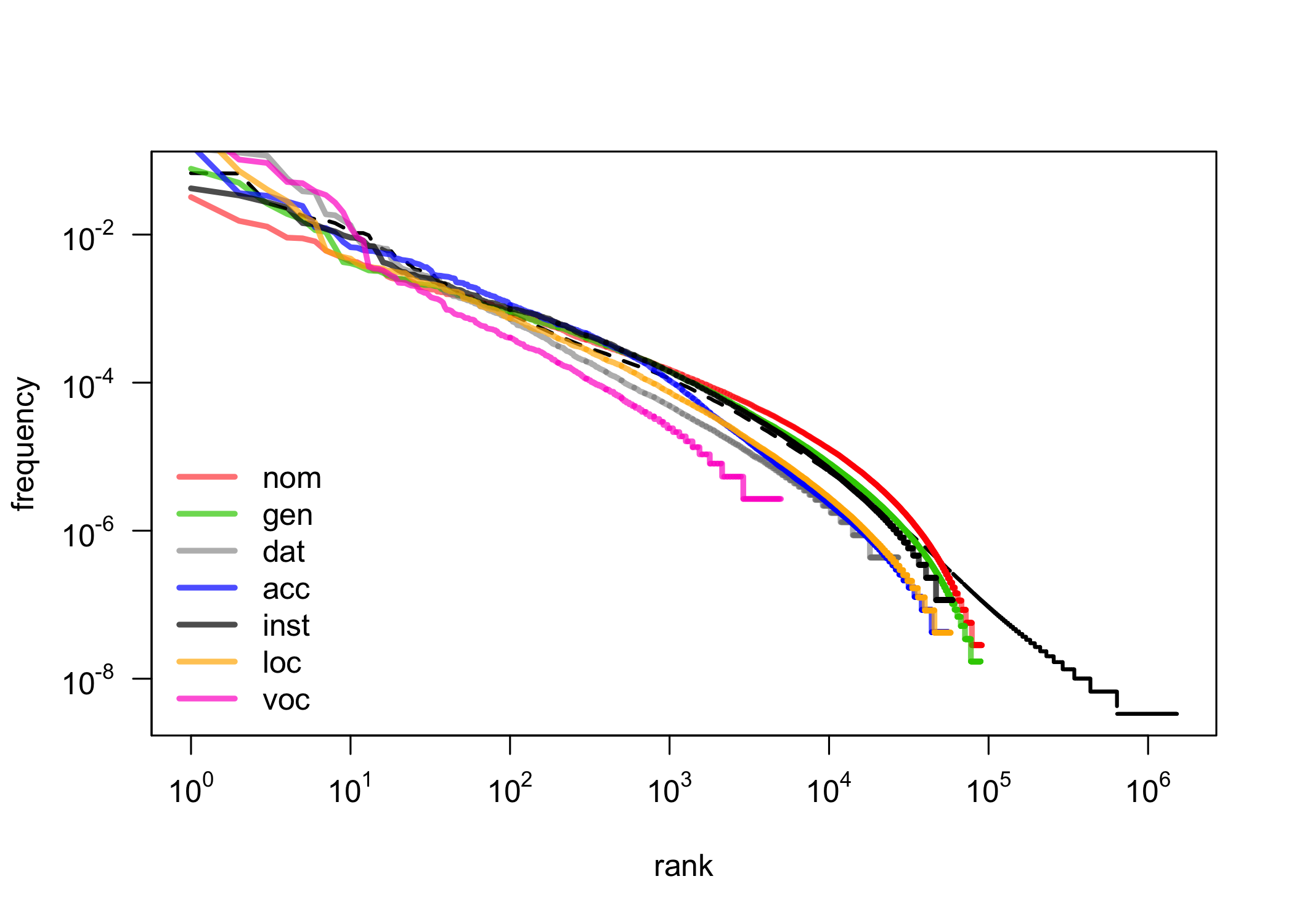## First observations: POS-tag ngrams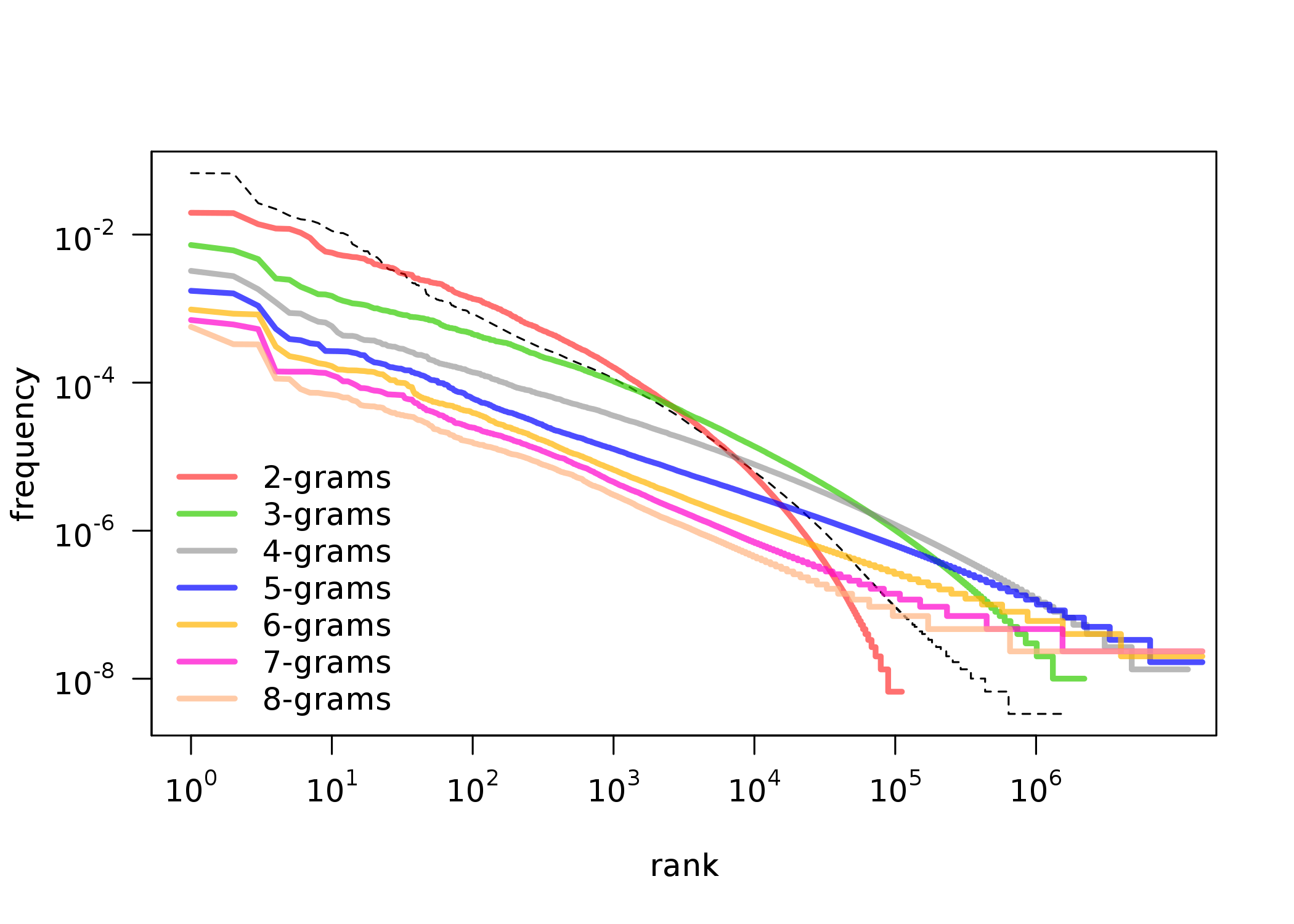## Modeling a power law distribution

• Since linear regression is simple to apply:
model = lm( var_frequency ~ var_rank )
• Maybe it could be applied to a log-transformed dataset?
model = lm( log(var_frequency) ~ log(var_rank) )

## Linear regression on log-log data

If in so doing one discovers a distribution that approximately falls on a straight line, then one can, if one is feeling particularly bold, assert that the distribution follows a power law, with a scaling parameter α given by the absolute slope of the straight line.

(Clauset et al., 2009)

## Fitting a power law

• Maximum likelihood estimators (MLEs) for continuous datasets

$\alpha = 1 + n \Big[ \sum_{i=1}^{n} \ln \frac{x_i}{x_{min}} \Big] ^{-1}$

• MLEs for discrete datasets:

$\alpha \simeq 1 + n \Big[ \sum_{i=1}^{n} \ln \frac{x_i}{x_{min} - \frac{1}{2}} \Big] ^{-1}$

• $$x_{min}$$ is estimated using the Kolmogorov-Smirnov (KS) statistic:

$D = \max_{x \geq x_{min}} | S(x) - P(x) |$

## Fitted parameters: $$x_{min}$$ (cutoff)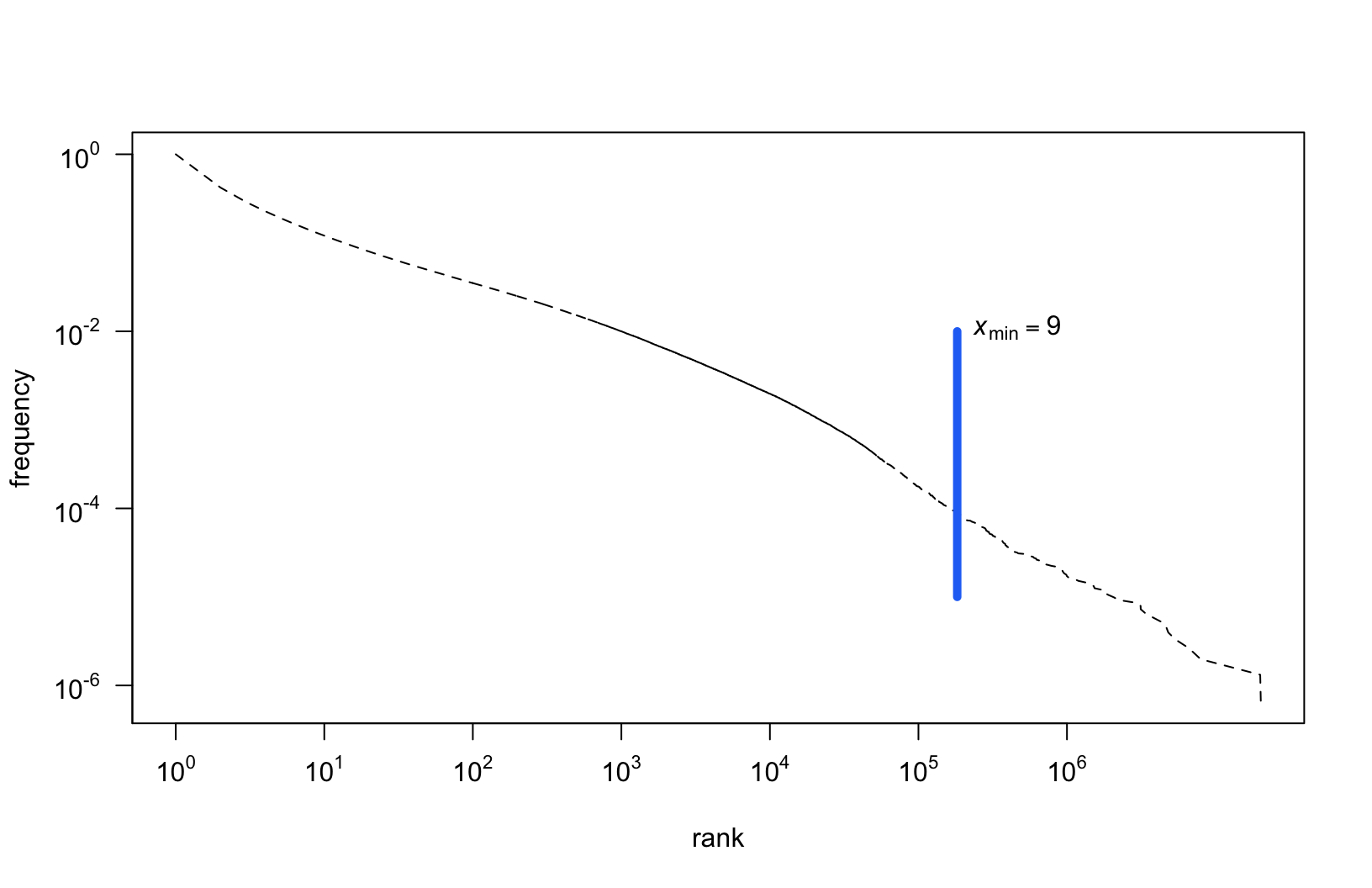## Fitted parameters: $$\alpha$$ (scaling)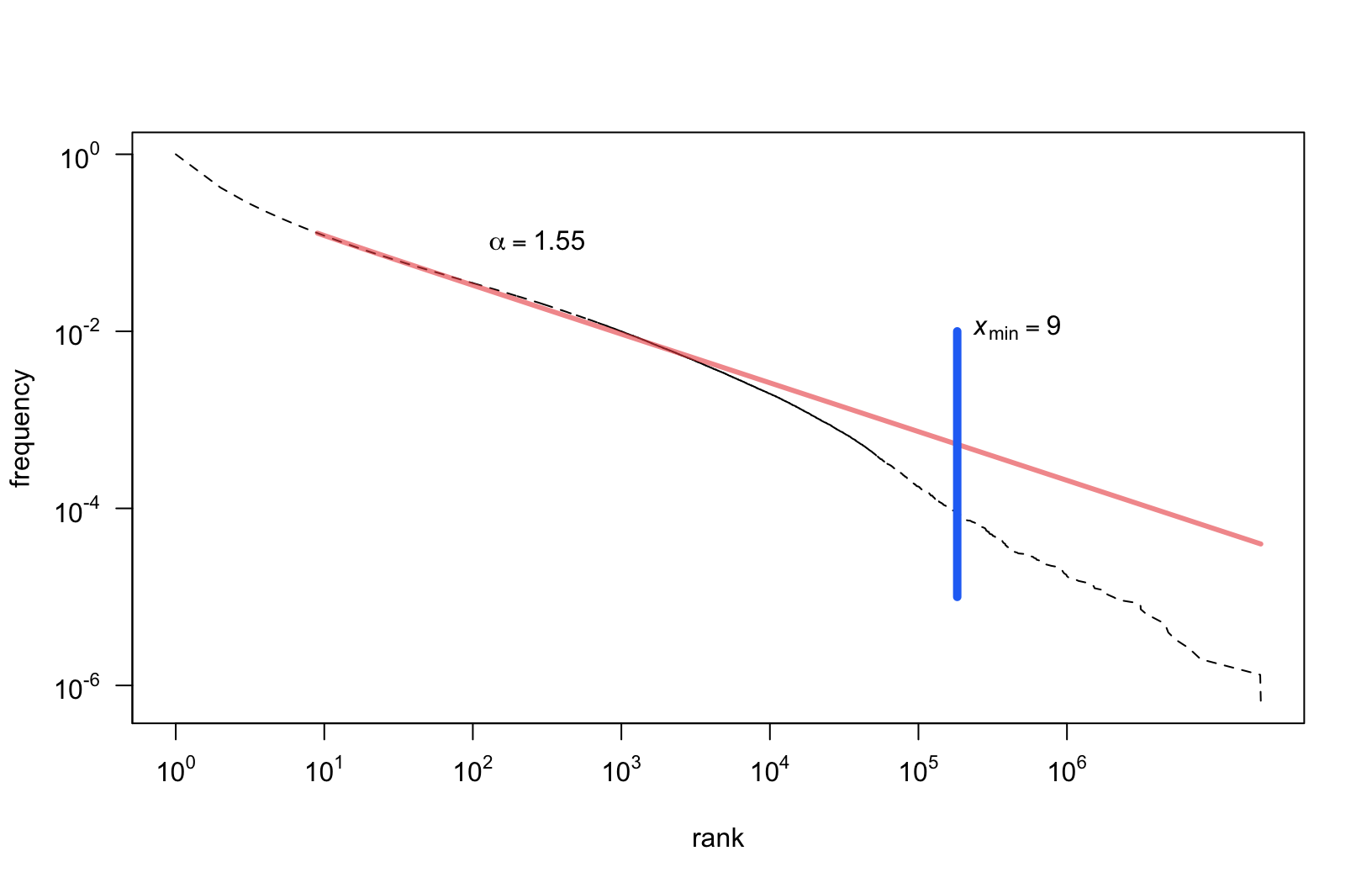## Results

• We fitted power law parameters for different categories:
• grammatical classes (parts of speech)
• inflection categories (cases, persons, numbers)
• POS-tags combined in 2-grams, 3-grams, …, 8-grams
• We compared $$\alpha$$ (scaling) of the estimated models
• We compared the proportion of observations above $$x_{min}$$

## What is perfectly Zipfian?

Prepositions and conjuctions:

POS Occurrences $$\alpha$$ ZTypes ZTokens
prep 28,787,398 1.14 97.9% 99.99%
conj 10,455,657 1.21 81.03% 99.99%
comp 4,145,149 1.2 87.23% 99.99%
• Short, closed classes
• Non-Zipfian elements include archaic vocabulary, present but underrepresented in relation to the whole corpus.

## What is least Zipfian?

Participles:

POS Occurrences $$\alpha$$ ZTypes ZTokens
praet 11,995,036 1.97 5.12% 81.23%
pant 35,235 2.05 14.54% 79.09%
ppas 3,187,531 2.24 4.62% 68.1%
pact 1,209,948 2.25 3.5% 65.32%
pcon 662,548 2.3 4.5% 64.38%
• Why? They’re very productive!

## Major parts of speech

• Subject and verb:
• Tokens - over 99% and 95% respectively
• Types - both only 17%
• Tokens - 94% and 98%
• Types - 10% and 18%
• These are also very productive categories!

## Cases

Stable, (un)expected Zipf results for both types and tokens:

Case ZTypes ZTokens
acc 98% 18%
voc 95.5% 10%
dat 93% 7%
loc 91% 3.5%
gen 88% 7%
inst 75% 2.5%
nom 65% 2.5%

## Relation between $$\alpha$$ (scaling) and coverage?

• Is there any relation between the parameter $$\alpha$$ (or slope of the model) and the number of observations above the $$x_{min}$$ cutoff point?
• To address it, we modeled:
• the relation between $$\alpha$$ and the proportion of tokens $$\geq x_{min}$$
• the relation between $$\alpha$$ and the proportion of types $$\geq x_{min}$$

## Parameter $$\alpha$$ vs. $$\sqrt{\%}$$ of Zipfian tokens## Parameter $$\alpha$$ vs. $$\%$$ of Zipfian types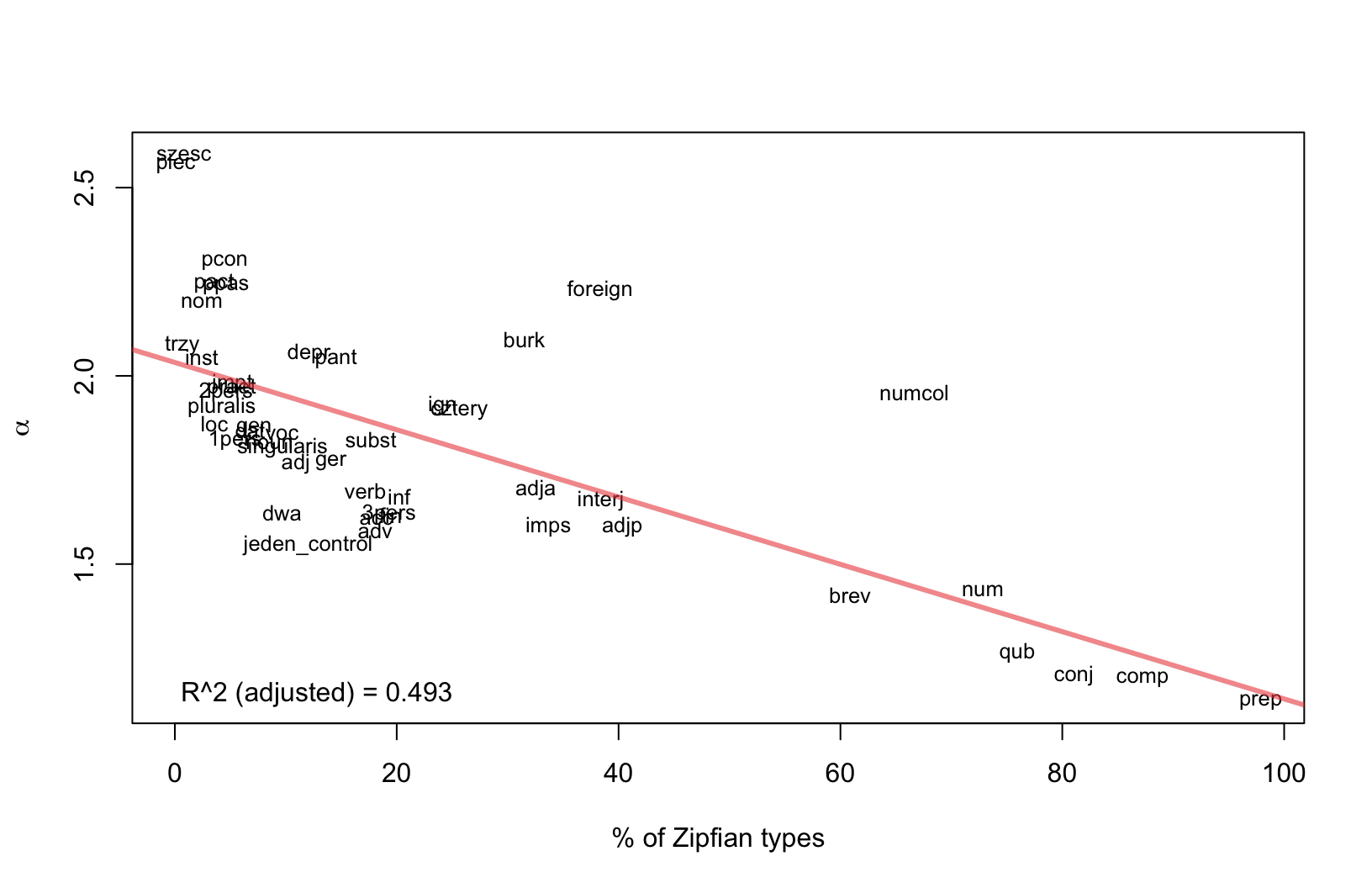## Conclusions

• Unzipped language categories do not necessarily follow a power law distribution.
• Productivity of a class seems to be responsible for a heavy tail.
• Classes of relatively low productivity (voc, praep) do follow Zipf’s law.
• The relation between $$\alpha$$ (the scaling parameter) and the proportion of a class following Zipf’s distribution worth further exploration.

## Thank you!

This research is part of project UMO-2013/11/B/HS2/02795, supported by Poland’s National Science Centre.